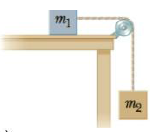Chapter 4, Problem 65P

Chapter
Section
Textbook Problem

Objects with masses m1 = 10.0 kg and m2 = 5.00 kg are connected by a light string that passes over a frictionless pulley as in Figure P4.64. If, when the system starts from rest, m2 falls 1.00 m in 1.20 s, determine the coefficient of kinetic friction between raj and the table.To determine
The co-efficient of kinetic friction.

Explanation

Given info: The masses are m1 = 10.00 kg and m2 = 5.0 kg. The mass m2 falls 1.00 m in 1.20 s.

From Newton’s equation of motion, the displacement is,

s=ut+12at2

• u is the initial velocity.
• t is the time taken.

Substitute 0 m/s for u in the above expression and re-arrange to get a.

a=2st2

Substitute 1.00 m for s and 1.20 s for t in the above expression to get a.

a=2(1.00m)(1.20s)2=1.39ms2

The free body diagram is given below.

From the free body diagram,

N=m1g (I)

m2gT=m2a (II)

TFk=m1a (III)

• m1 and m2 are the masses of blocks.
• a is the acceleration.
• g is the acceleration due to gravity.
• T is the tension in the cable.
• Fk is the kinetic friction force.

Add Equations (II) and (III).

m2gFk=a(m1+m2)

On Re-arranging,

Fk=m2(ga)m1a

Substitute 10

Still sussing out bartleby?

Check out a sample textbook solution.

See a sample solution

The Solution to Your Study Problems

Bartleby provides explanations to thousands of textbook problems written by our experts, many with advanced degrees!

Get Started